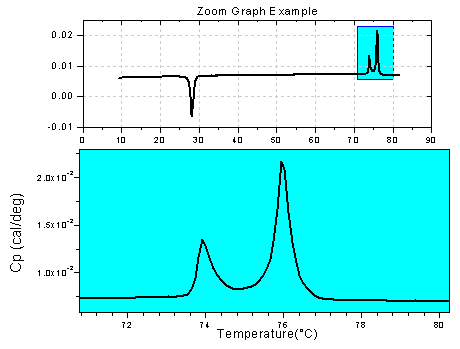Skip Navigation Links.

### Zoom Graph with Line PlotThis graph is an example of Origin's Zoom Graph plot template. This interactive, two layer graph window, plots your data as a line plot in both layers. A movable rectangle is included. This rectangle can be placed over an area of interest in the plot. The data contained in the rectangle is then displayed in the lower panel so that you can get a closer look. In this example a series of peaks is highlighted.

 © OriginLab Corporation. All rights reserved.× _ Let's Chat